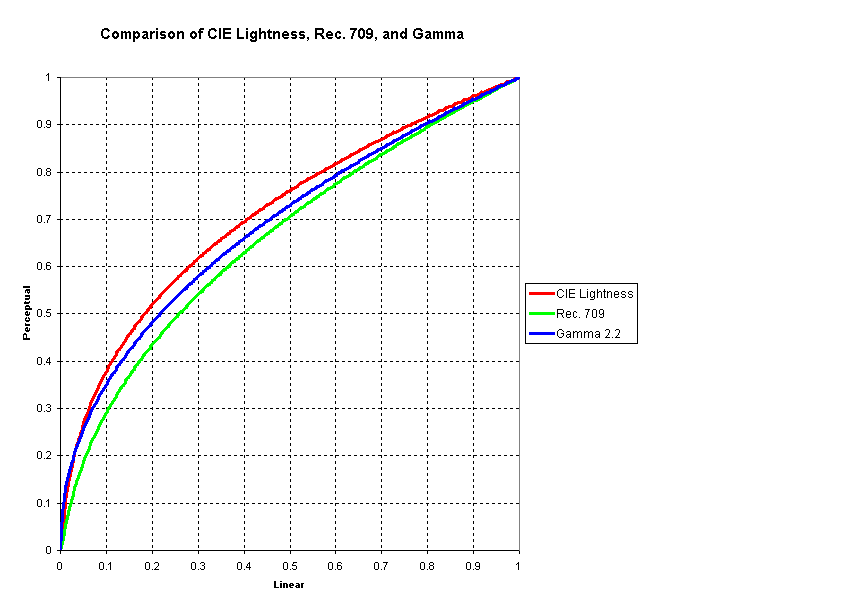Psychometric Lightness and Gamma
Prepared 2005-03-26 by Bill Claff

### CIE 1931

As part of their landmark work in 1931 the CIE determined a formula for psychometric lightness. The CIE formula for lightness is:

 L* = 903.3 ∙ (Y / Yn) (Y/ Yn) ≤ 0.008856 L* = 116 ∙ (Y / Yn)1/3 – 16 (Y/ Yn) > 0.008856

Where Y is luminance, Yn is the luminance of the white reference. This produces as L* value between 0 and 100.

The exact crossover point and slope are:

 (Y / Yn) = (6 / 29)3 = 0.00885645 Slope = 8 / (6 / 29)3 = 903.296

So L* is a straight line from 0 to 8 and a ⅓ power function from 8 to 100.

It is straightforward to invert these equations and compute Y as a function of L* and Yn:

 Y = (L* / 902.3) ∙ Yn L* ≤ 8 Y = ((L* + 16) / 116)3 * Yn L* > 8

Notice that Y = .184 for L* = 50 and Yn = 1; this is where the 18% gray standard originates.

### Rec. 709

In video systems lightness is approximated by a .45 power function. This is formalized in Rec. 709 as:

 R’709 = 4.5 ∙ R R ≤ 0.018 R’709 = 1.099 ∙ R0.45 – 0.099 R > 0.018

Where R is linear‑light intensity.

The inverse of these equations is:

 R = R’709 / 4.5 R’709 ≤ 0.081 R = ((R’709 + 0.099) / 1.099)1/0.45 R’709 > 0.081

The constraints are exact and derive from:

 (0.099 / 5.5) = 0.018 (0.099 / 5.5) ∙ 4.5 = 0.081

### Gamma

In a video display system intensity is related to voltage according to the formula:

 I = Vg

Where the Greek letter gamma g has the value 2.5. As a practical matter, due to viewing conditions, this 2.5 value is typically under corrected as 2.2 (for NTSC, other video standards are different) and the Rec. 709 formulas are approximated as:

 R’ = R(1/g) R = (R’)g

Where g is 2.2.

These power functions, with a gamma of 2.2, are frequently used to map between linear and perceptual values. Although the “gamma function” is commonly used it is arguable that CIE Lightness is the more precise formulation; and in fact CIE Lightness is the formula use for L in the CIE Lab color model rather than a gamma function.

Note that applying a gamma function with a gamma of 2.2 to the 18% gray value that derived from CIE Lightness of 50% (L* = 50) results in a value of 46% not 50%.

To achieve 50% requires a 21.8% gray value corresponding to a CIE Lightness of 53.78% (L* = 54). This is where the Adobe L54 value comes from.

### Comparison Chart

For reference here is a chart comparing the CIE Lightness, Rec. 709, and Gamma transfer functions: